# 算法设计：两种快速排序代码实现

## 快排的大致思想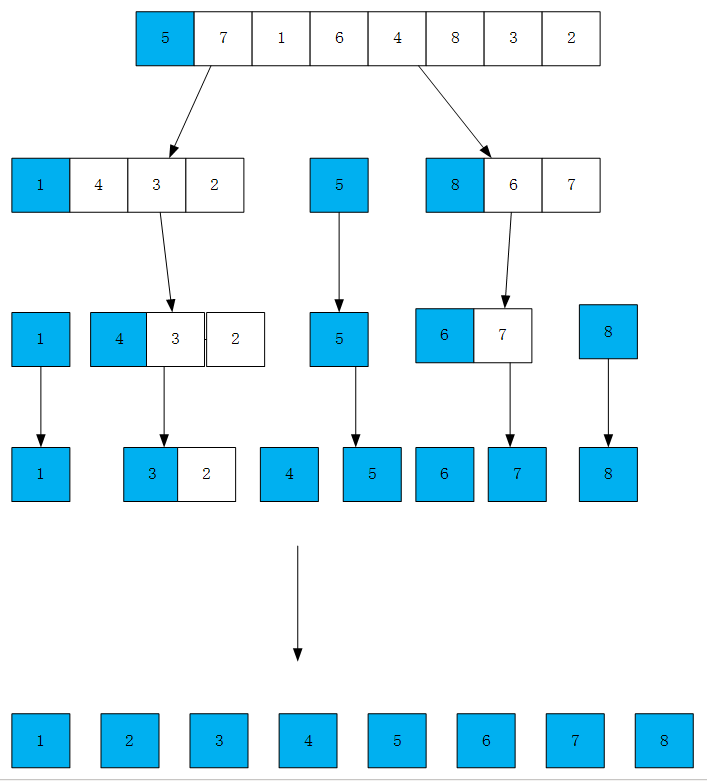## split算法解析

split算法使用一个单向的指针来对数组进行遍历，首先将数组首元素设置为比较元素，然后将第二个开始的元素依次与比较元素比较，如果大于比较元素则跳过，如果小于比较元素，则将其与前面较大的元素进行交换，将数组中所有元素交换完毕后，再将比较元素放到中间位置。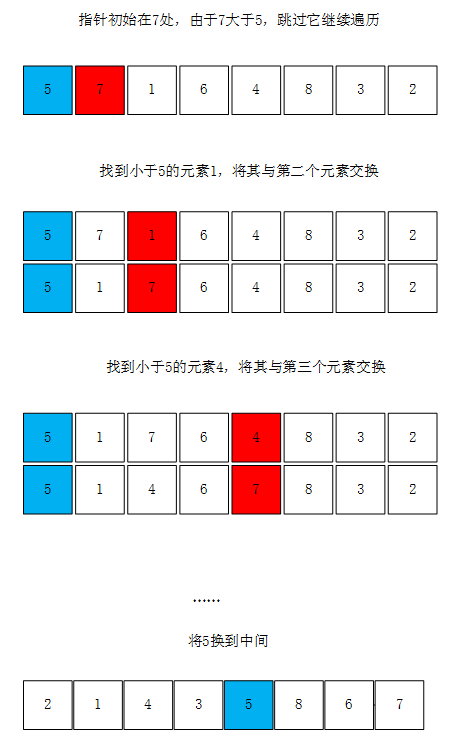### split算法实现（c）：

 1 //划分数组的函数
2 int split(int a[], int low, int high)
3 {
4     int i = low;    //i指向比较元素的期望位置
5     int x = a[i];    //将该数组第一个元素设置为比较元素
6     //从数组的第二个元素起开始遍历，若找到的元素大于比较元素，则跳过
7     for(int j = low+1;j<=high;j++)
8         //若找到了小于比较元素的数，则将其与前面较大的数进行交换
9         if (a[j] <= x)
10         {
11             i++;
12             swap(a[i], a[j]);
13         }
14     swap(a[low], a[i]);    //将比较元素交换到期望位置
15     return i;
16 }

### split算法实现（java）：

 1 //划分数组
2     public static int split(int a[], int low, int high)
3     {
4         int i = low;    //i指向比较元素的期望位置
5         int x = a[low];    //将该组的第一个元素作为比较元素
6         //从第二个元素开始，若当前元素大于比较元素，将其跳过
7         for(int j = low+1; j <= high; j++)
8             //若找到了小于比较元素的元素，将其与前面较大的元素进行交换
9             if(a[j] <= x)
10             {
11                 i++;
12                 if(i != j)
13                     swap(a, i, j);
14
15             }
16         swap(a, i, low);     //将比较元素交换到正确的位置上
17         return i;    //返回比较元素的位置
18     }

## partition算法解析

partition算法使用头尾两个方向相反的指针进行遍历，先将数组第一个元素设置为比较元素，头指针从左至右找到第一个大于比较元素的数，尾指针从右至左找到第一个小于比较元素的数，全部交换完毕后将比较元素放到中间位置。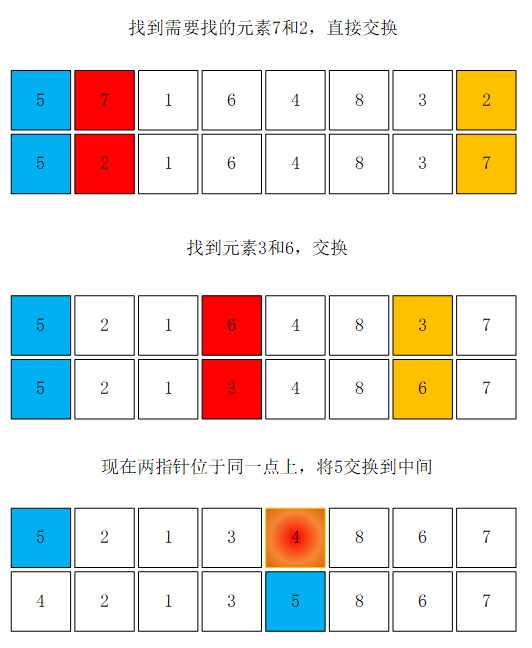### partition算法实现（c）：

 1 int partition(int a[], int low, int high)
2 {
3     int x = a[low];    //将该数组第一个元素设置为比较元素
4     int i = low;    //指向数组头的指针
5     int j = high;    //指向数组尾的指针
6     while (i < j)
7     {
8         while (i < j && a[j] >= x)
9             j--;    //从右至左找到第一个小于比较元素的数
10         while (i < j && a[i] <= x)
11             i++;    //从左至右找到第一个大于比较元素的数
12         /*需要注意的是，这里的j--与i++的顺序不可以调换！
13           如果调换了顺序，i会走过头，以至于将后面较大的元素交换到数组开头*/
14
15         //将大数与小数交换
16         if (i != j)
17             swap(a[i], a[j]);
18     }
19     swap(a[low], a[i]);    //将比较元素交换到期望位置
20     return i;    //返回比较元素的位置
21 }

### partition算法实现（java）：

 1     //划分数组
2     public static int partition(int a[], int low, int high)
3     {
4         int x = a[low];    //将该数组第一个元素设置为比较元素
5         int i=low;
6         int j=high;
7         while(i < j)
8         {
9             while(i<j && a[i] <= x)
10                 i++;
11             while(i<j && a[j] >= x)
12                 j--;
13
14
15             if(i!=j)
16                 swap(a, i, j);
17         }
18         swap(a, j, low);
19         return j;
20     }

## 两种算法的完整代码和实际演示

### split算法（c）：

 1 #include<stdio.h>
2
3 void swap(int &a, int &b)
4 {
5     int t = a;
6     a = b;
7     b = t;
8 }
9
10 //划分数组的函数
11 int split(int a[], int low, int high)
12 {
13     int i = low;    //i指向比较元素的期望位置
14     int x = a[i];    //将该数组第一个元素设置为比较元素
15     //从数组的第二个元素起开始遍历，若找到的元素大于比较元素，则跳过
16     for(int j = low+1;j<=high;j++)
17         //若找到了小于比较元素的数，则将其与前面较大的数进行交换
18         if (a[j] <= x)
19         {
20             i++;
21             swap(a[i], a[j]);
22         }
23     swap(a[low], a[i]);    //将比较元素交换到期望位置
24     return i;
25 }
26
27 //快速排序
28 void quicksort(int a[], int low, int high)
29 {
30     if (low < high)
31     {
32         int i = split(a, low, high);    //划分数组并获得比较元素位置
33         quicksort(a, low, i - 1);    //对比较元素左边进行排序
34         quicksort(a, i + 1, high);    //对比较元素右边进行排序
35     }
36 }
37
38 int main()
39 {
40     int a[] = { 5,7,1,6,4,8,3,2 };
41     int length = sizeof(a) / sizeof(a);
42     quicksort(a, 0, length - 1);
43     for (int i = 0; i < length; i++)
44         printf("%d ", a[i]);
45     printf("\n");
46     return 0;
47 }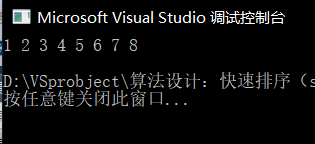### split算法（java）：

 1 //快速排序split实现方法
2 public class T1 {
3     public static void main(String args[])
4     {
5         int a[] = {5,7,1,6,4,8,3,2};
6         quickSort(a, 0, a.length-1);
7         for(int i=0;i<a.length;i++)
8             System.out.print(a[i] + " ");
9         System.out.println();
10     }
11
12     //交换方法
13     public static void swap(int a[], int i, int j)
14     {
15         int t = a[i];
16         a[i] = a[j];
17         a[j] = t;
18     }
19
20     //划分数组
21     public static int split(int a[], int low, int high)
22     {
23         int i = low;    //i指向比较元素的期望位置
24         int x = a[low];    //将该组的第一个元素作为比较元素
25         //从第二个元素开始，若当前元素大于比较元素，将其跳过
26         for(int j = low+1; j <= high; j++)
27             //若找到了小于比较元素的元素，将其与前面较大的元素进行交换
28             if(a[j] <= x)
29             {
30                 i++;
31                 if(i != j)
32                     swap(a, i, j);
33
34             }
35         swap(a, i, low);     //将比较元素交换到正确的位置上
36         return i;    //返回比较元素的位置
37     }
38
39     public static void quickSort(int a[], int low, int high)
40     {
41         if(low < high)
42         {
43             int i = split(a, low, high);    //划分并获取比较元素的位置
44             quickSort(a, low, i-1);     //对比较元素左边的数组进行排序
45             quickSort(a, i+1, high);     //对比较元素右边的数字进行排序
46         }
47     }
48 }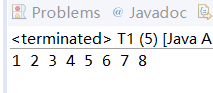### partition算法（c）：

 1 #include<stdio.h>
2
3 //交换函数
4 void swap(int &a, int &b)
5 {
6     int t = a;
7     a = b;
8     b = t;
9 }
10
11 int partition(int a[], int low, int high)
12 {
13     int x = a[low];    //将该数组第一个元素设置为比较元素
14     int i = low;    //指向数组头的指针
15     int j = high;    //指向数组尾的指针
16     while (i < j)
17     {
18         while (i < j && a[j] >= x)
19             j--;    //从右至左找到第一个小于比较元素的数
20         while (i < j && a[i] <= x)
21             i++;    //从左至右找到第一个大于比较元素的数
22         /*需要注意的是，这里的j--与i++的顺序不可以调换！
23           如果调换了顺序，i会走过头，以至于将后面较大的元素交换到数组开头*/
24
25         //将大数与小数交换
26         if (i != j)
27             swap(a[i], a[j]);
28     }
29     swap(a[low], a[i]);    //将比较元素交换到期望位置
30     return i;    //返回比较元素的位置
31 }
32
33 void quicksort(int a[], int low, int high)
34 {
35     if (low < high)
36     {
37         int i = partition(a, low, high);    //划分数组并获取比较元素的位置
38         quicksort(a, low, i - 1);    //对比较元素左边进行排序
39         quicksort(a, i + 1, high);    //对比较元素右边进行排序
40     }
41 }
42
43 int main()
44 {
45     int a[] = { 5,7,1,6,4,8,3,2 };
46     int length = sizeof(a) / sizeof(a);
47     quicksort(a, 0, length - 1);
48     for (int i = 0; i < length; i++)
49         printf("%d ", a[i]);
50     printf("\n");
51     return 0;
52 }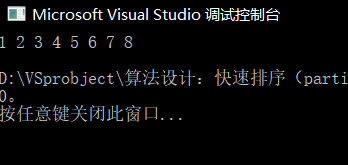### partition算法（java）：

 1 //快速排序partition实现方法
2 public class T2 {
3     public static void main(String args[])
4     {
5         int a[] = {5,7,1,6,4,8,3,2};
6         quicksort(a, 0, a.length-1);
7         for(int i=0;i<a.length;i++)
8             System.out.print(a[i] + " ");
9         System.out.println();
10     }
11
12     public static void swap(int a[], int i, int j)
13     {
14         int t = a[i];
15         a[i] = a[j];
16         a[j] = t;
17     }
18
19     //划分数组
20     public static int partition(int a[], int low, int high)
21     {
22         int x = a[low];    //将该数组第一个元素设置为比较元素
23         int i=low;
24         int j=high;
25         while(i < j)
26         {
27             while(i<j && a[j] >= x)
28                 j--;     //从右至左找到第一个小于比较元素的数
29             while(i<j && a[i] <= x)
30                 i++;     //从左至右找到第一个大于比较元素的数
31             /*需要注意的是，这里的j--与i++的顺序不可以调换！
32              *如果调换了顺序，i会走过头，以至于将后面较大的元素交换到数组开头*/
33
34             //将大数与小数交换
35             if(i!=j)
36                 swap(a, i, j);
37         }
38         swap(a, i, low);    //将比较元素交换到期望位置
39         return i;     //返回比较元素的位置
40     }
41
42     public static void quicksort(int a[], int low, int high)
43     {
44         if(low < high)
45         {
46             int i = partition(a, low, high);    //划分数组并获取比较元素的位置
47             quicksort(a, low, i-1);     //对比较元素左边进行排序
48             quicksort(a, i+1, high);     //对比较元素右边进行排序
49         }
50     }
51 }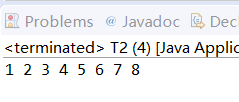posted @ 2018-11-23 21:43  Sunrise_1018  阅读(17846)  评论(3编辑  收藏